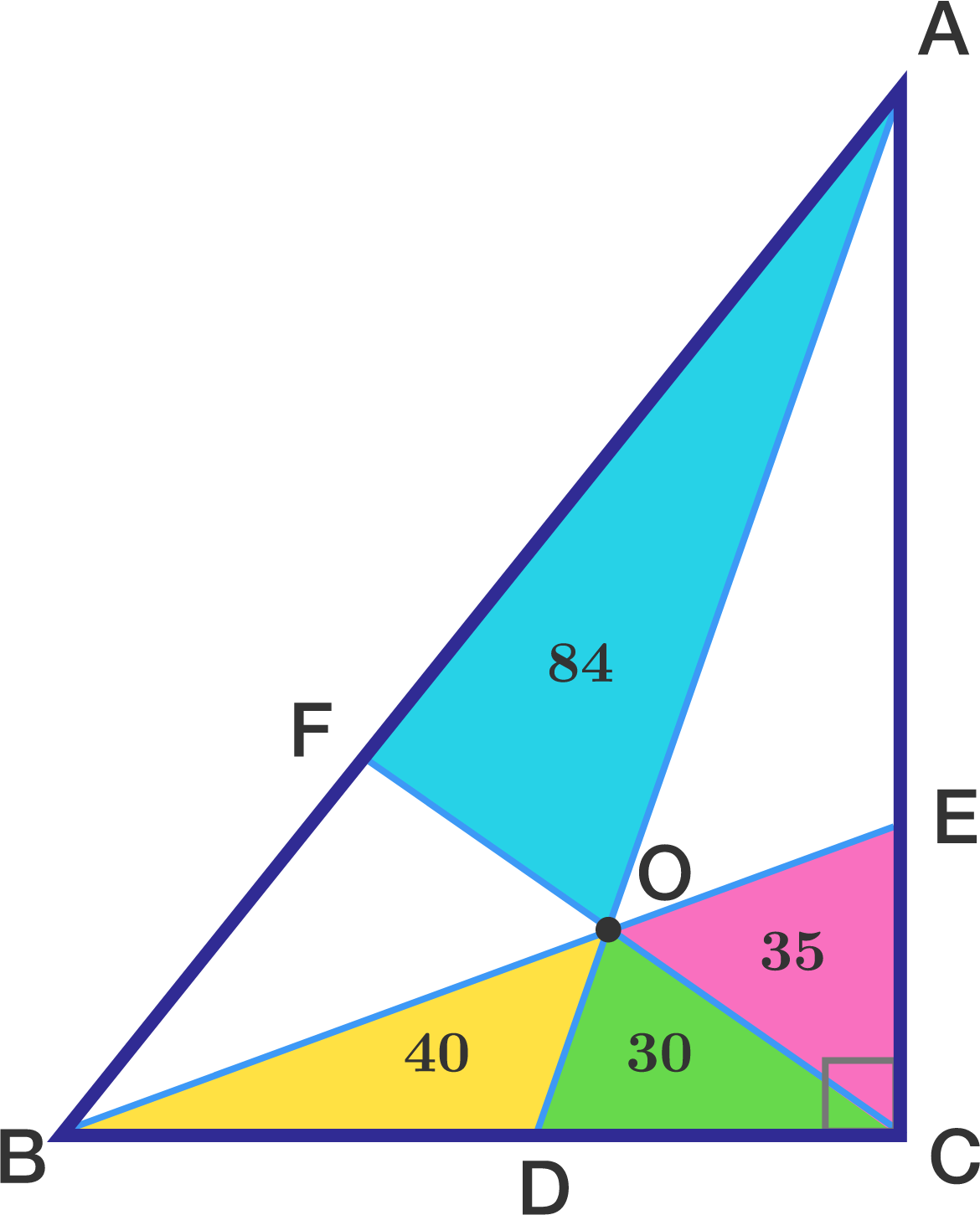# Partitioned Areas

Geometry Level 2A right triangle $ABC$ is divided into six smaller triangles by lines drawn from the vertices through a common interior point named as $O$.

These lines which are drawn from vertices $A,B,C$ meet $BC,AC,AB$ at points $D,E,F$, respectively.

The areas of triangles $AFO, OEC, ODC, OBD$ are 84,35,30 and 40, respectively.

What is the area of triangle $ABC$?

×